# Exciton Quasimolecules and Exciton Quasicrystals: Theory

Sergey I Pokutnyi*

Department of Theoretical Physics of Nano systems, Chuіko Institute of Surface Chemistry of National Academy of Sciences of Ukraine, Ukraine

Corresponding Author

Received Date: November 13, 2019  Published Date: December 02, 2019

#### Abstract

In review, deals with the theory of exciton Quasimolecules in a Nano heterostructures. It has been found that the formation of a exciton Quasimolecules in a Nano heterostructures made up of aluminum oxide quantum dots synthesized in a dielectric matrix is of threshold character and can occur in a Nano systems where the distance D between the surfaces of quantum dots is given by the condition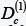The existence of such distancearises from quantum size effects in which the decrease in the energies of interaction of the electrons and holes entering into the Hamiltonian of the “exciton molecule” with decrease of the distance D between the surfaces of the QD cannot compensate for the increase in the kinetic energy of the electrons and holes. At larger distances D between the surfaces of quantum dots:the biexciton breaks down into two excitons (consisting of spatially separated electrons and holes), localized over QD surfaces.

It was shown that the convergence of two quantum dots up to a certain critical value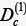between surfaces of quantum dot lead to overlapping of electron orbitals of super atoms and the emergence of exchange interactions. In this case the overlap integral of the electron wave functions takes a significant value. As a result, the conditions for the formation of quasi-molecules from quantum dots can be created.

We have shown that in such a Nano heterostructures acting as “exciton molecules” (biexcitons consisting of spatially separated electrons and holes) are the quantum dots of aluminum oxide with excitons localizing over their surfaces. The position of the biexciton state energy band depends both on the mean radius of the quantum dots, and the distance between their surfaces, which enables one to purposefully control it by varying these parameters of the nanostructure.

As our variational calculations show, the interaction of the excitons with the surfaces of quantum dots (“intramolecular” interaction) is much stronger than that between quantum dots (“intermolecular” interaction). Due to the translational symmetry of such a Nano heterostructures of quantum dots, it permits propagation of electronic excitation in the form of biexcitons.

As follows form the results of the variational calculations, the major contribution to the biexciton binding energy is from the energy of exchange interaction of electrons and holes, which by far surpasses that from their Coulomb interaction.

It is established that at constant concentrations of biexcitons at temperatures T below a certain critical temperature Tc due to the radiative annihilation of one of the excitons forming a biexciton one can expect a new spectral band of luminescence shifted relative to the exciton band by the biexciton binding energy Eв. This new luminescence band disappears at temperatures above Tc. At a constant temperature Т

Keywords: Electrons; Holes; Biexciton ground singlet states; Binding energy; Coulomb; Polarization and exchange interaction; Quantum dots; Nano heterostructures

#### Introduction

Recent advances of solid-state technology made it possible to produce a nano heterostructures (NHS), where acting as lattice points are spherical dielectric or semiconductor quantum dots (QDs) with the mean radius a =1-10 nm implanted in a transparent dielectric (or semiconductor) matrix . Such sizes of QD are comparable with the de Broglie wavelength of the electron and hole or (and) with their Bohr radii. This leads to the spatial dimensional quantization of charge carriers playing a substantial part in optical and electro-optical processes in such nano systems [1-24].

The studies of quasi 0-sD nano systems made up of spherical QD with the mean radius in the range of 1 to 10 nm consisting of semiconductor (cadmium sulfide and selenide, gallium arsenide, zinc selenide) [1-3] and dielectric (aluminum oxide) [4-6] materials synthesized in dielectric (semiconductor) matrices attract considerable interest due to their unique photoluminescence properties, ability to effectively emit light in the visible and near infrared spectral range at room temperatures [1-6]. Optical and electro-optical properties of such quasi 0-D nano systems are to a large extent governed by the energy spectrum of the spatially confined electron-hole pair (exciton) [1-24].

During investigation of the optical characteristics of nano systems with CdS, ZnSe, Al2O3 and Ge quantum dots in experimental papers [11-15] it was found that the electron can be localized above the surface of the QD while the hole here moves in the volume of the QD. In [25-29] the appearance of super atoms located in dielectric matrices as cores containing CdS, ZnSe, Al2O3 and Ge quantum dots was apparently established experimentally for the first time. A substantial increase in the bond energy of the ground state of an electron in a super atom in comparison with the bond energy of an exciton in CdS, ZnSe and Al2O3 and single crystals was detected in .

In [26-29] the optical characteristics of samples of borosilicate glasses doped with CdS, ZnSe and Al2O3 at concentrations between x≈0.003% to 1% were investigated. The average radii ā of CdS and ZnSe QDs were in the range of ā≈2.0-20 nm. When there were large concentrations of CdS quantum dots in the samples (from x≈0.6% to x≈1%) a maximum, interpreted by the appearance of bonded QD states, was detected in the low-temperature absorption spectra. In order to explain the optical characteristics of such nano systems we proposed a model of a quasimolecule representing two ZnSe and CdS QDs that form an exciton quasimolecule as a result of the interaction of electrons and holes.

It was noted [25,27] that, at such a QD content in the samples, one must consider the interaction between charge carriers localized above the QD surfaces. Therefore, in [10,15], we develop the theory of an exciton quasimolecule (or biexciton) (formed from spatially separated electrons and holes) in a nano system that consists of ZnSe and CdS QDs synthesized in a borosilicate glassy matrix. Using the variational method, we obtain the total energy and the binding energy of the exciton quasimolecule (or biexciton) singlet ground state in such system as functions of the spacing between the QD surfaces and of the QD radius. We show that the biexciton formation is of the threshold character and possible in a nano system, in which the spacing between the QD surfaces exceeds a certain critical spacing. It is established that the spectral shift of the low-temperature luminescence peak  in such a nano system is due to quantum confinement of the energy of the biexciton ground state.

The convergence of two (or more) QDs up to a certain critical value Dc between surfaces of QD lead to overlapping of electron orbitals of super atoms and the emergence of exchange interactions . In this case the overlap integral of the electron wave functions takes a significant value. As a result, the conditions for the formation of quasi-molecules from QDs can be created . One can also assume that the above conditions of formation of quasimolecules can be provided by external physical fields. This assumption is evidenced by results of [30,31], in which the occurrence of the effective interaction between QDs at considerable distances under conditions of electromagnetic field was observed experimentally. In  energies of the ground state of “vertical” and “horizontal” located pair of interacting QDs (“molecules” from two QDs) were determined as a function of the steepness of the confining potential and the magnetic field strength. The quantum part of nano computer, which was implemented on a pair of QDs (“molecules” from two QDs) with charge states is n qubits . The first smoothly working quantum computer has been on QDs with two electron orbital states as qubits, described by a pseudospin (½). As a single cell was taken a couple of asymmetric pair QDs with different sizes and significantly different own energy. The electron injected into the heterostructure from the channel occupied the lower level. That is, it was in a QD with larger size.

In the present review we show that the biexciton formation in a NHS made up of aluminum oxide quantum dots synthesized in a dielectric matrix is of threshold character and can occur in a nano system where the distance D between the surfaces of QD is given by the condition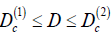We also demonstrate that in such NHS acting as “exciton molecules” (or biexciton) are the quantum dots of aluminum oxide with excitons localizing over their surfaces. The position of the biexciton state energy band is shown to depend both on the mean radius of quantum dots, and the distance between their surfaces, which enables one to purposefully control it by varying these parameters of the NHS. It is established that at constant concentrations of biexcitons at temperatures T below a certain critical temperature Tc due to the radiative annihilation of one of the excitons forming a biexciton one can expect a new spectral band of luminescence shifted relative to the exciton band by the biexciton binding energyEa. This new luminescence band disappears at temperatures above Tc. At a constant temperature Т

#### Energy of the Exciton Quasimolecules Ground Singlet State Formed from Spatially Separated Electrons and Holes

Let us consider a model of a nano system composed of two dielectric (semiconductor) spherical quantum dots QD(A) and QD(B) of radius а synthesized in a dielectric matrix with permittivity ε1 (D is the distance between the spherical surfaces of QD). Quantum dots are of a dielectric (semiconductor) material with permittivity ε2. For simplicity and without loss of generality let us assume that holes h(A) и h(B) with effective masses mh are located at the centers of QD (A) and QD (B) while electrons е(1) and е(2) with effective masses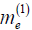are localized near the spherical surfaces of QD (A) and QD (B), respectively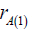the distance from the electron е(1) to the center of QD (A);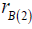the distance from the electron е(2) to the center of QD (B) (Figure 1). This assumption is justified by the fact thatLet us also infer that an infinitely high potential barrier exists on the spherical interface between the QD and the matrix so that the electrons cannot get into the quantum dot while the holes cannot escape from it.

Let us now use this model to consider the possibility of the formation of an exciton from spatially separated electrons and holes (the holes are located at the centers of QD(A) and QD(B) and electrons are localized near their spherical surfaces). Using adiabatic approximation and the effective mass approximation, the Hamiltonian of the biexciton (of spatially separated electrons and holes) can be written in the form :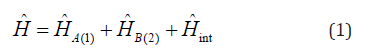where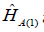and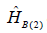are the Hamiltonians of the excitons of spatially separated hole h(A) and electron е(1) and hole h(B) and electron е(2), respectively. The contribution of the energy of polarization interaction with the surface of QD to the Hamiltonians of the excitons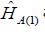andcan be, as a first approximation neglected . Thus, the exciton Hamiltonian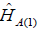takes the form :where the first term is the exciton kinetic energy operator and the energy of Coulomb interaction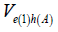between electron е(1) and hole h(A) is given be the following expression [7,8]:where Eg is the bandgap energy of the dielectric (semiconductor) with permittivity ε2.The Hamiltonian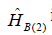is of the same form asIn the first approximation we can neglect the contributions to the Hamiltonian H ̂int of the interaction energies of the electrons e(1) and e(2) and the holes h(A) and h(B) with polarization fields induced by these charge carriers on the surfaces of QD (A) and QD (B) . Thus the Hamiltonian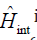incorporates only the energies of Coulomb interaction of electron e(1) with hole h(B), and electron е(2) with hole h(A), as well as that between electrons е(1) and е(2), and holes h(A) and h(B).

Under the assumption that the spins of the electrons е(1) and е(2) are antiparallel let us write down the normalized wave function of the ground singlet state of the biexciton as a symmetric linear combination of wave functions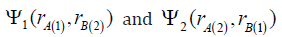: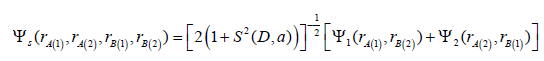where S (D, a) is the overlap integral of single-electron wave functions. Assuming that the electrons e(1) and e(2) move independently from each other, let us represent the wave functions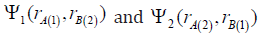as a product of single-electron wave functions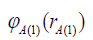as well as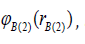and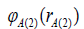respectively . Let us also represent the singleelectron wave functions as variational functions of Coulomb type :where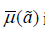is a variational parameter,is the Bohr radius of the 2D exciton localized over the flat interface between the semiconductor (dielectric) with permittivity ε2 and the matrix with permittivity ε1 (the hole is in the semiconductor and the electron is in the matrix, е is the electron charge,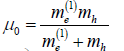is the reduced effective mass of the 2D exciton,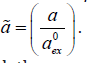In the framework of the variational method, the energy of the biexciton ground singlet state, as a first approximation, is given by the mean value of the Hamiltonian H (1) over the states described by the wave functions of the 0th approximation Ψs (4) :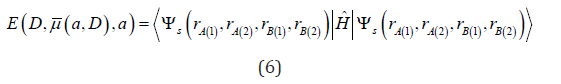As follows from (6), the total energy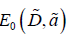of the biexciton ground singlet state takes the form :whereis the binding energy of the ground singlet state of the biexciton, andis the binding energy of the ground state of the exciton (consisting of spatially separated electron and hole) localized over the surface of QD, which was worked out in [7,8] (Figure 2)

Figure 2 presents the results of the variational calculations of the binding energystudied in [4-6] of the biexciton ground state in a nano system with QD of aluminum oxide of the mean radius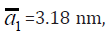(permittivity ε2 = 10 , effective mass of the hole in QD synthesized in a dielectric matrix of the vacuum oil VM-4 (permittivity ε1 = 1.96, the effective mass of the electron in the matrixwas deduced in  and amounts to 0,537, the Bohr radius (5а) of the 2D excitonAuthors of [4-6] studied nanostructures doped with х = 0.003% to 1% concentrations of aluminum oxide. At concentrations of QD higher than x ≅ 0.6% one needs to consider the interactions of the charges localized over the surfaces of quantum dots. The variational method that we used for the calculation of the biexciton ground state binding energy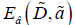is applicable provided that it is much smaller than the binding energy of the exciton ground state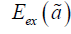i.e. the following condition must be fulfilled: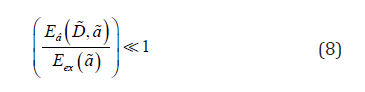The binding energyof the biexciton ground state in a nano system with QD of aluminum oxide of the mean radius=3.18 nm has a minimum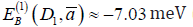(at the distance D1≅2.93 nm) (Figure 2)corresponds to the critical temperature Tс ≈ 81.6 K). As it follows form Figure 1, biexciton appears in the nano system at distances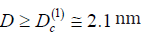between the surfaces of QD. The formation of such a biexciton (,,exciton molecule”) is of threshold character and can occur in a nano system with quantum dots of the mean radius a ̅1, where the distance D between the surfaces of QD exceeds a certain critical value (1) . The existenceof such distancearises from quantum size effects in which the decrease in the energies of interaction of the electrons and holes entering into the Hamiltonian (Eq. (1)) of the “exciton molecule” with decrease of the distance D between the surfaces of the QD cannot compensate for the increase in the kinetic energy of the electrons and holes.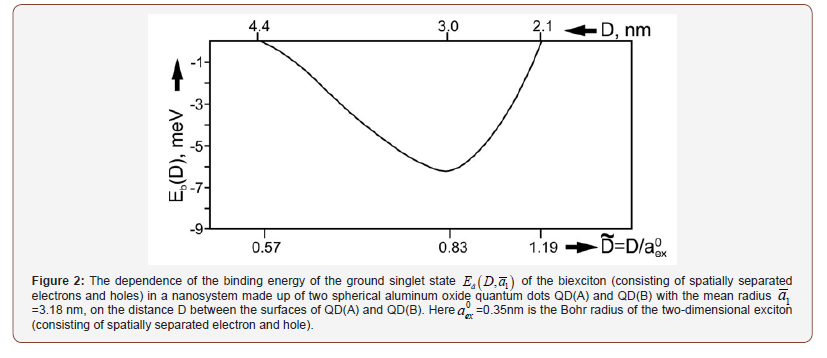The binding energy of the exciton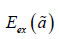amounts to≅-100.8 meV [7,8], with the energy of the biexciton ground state (7) taking the value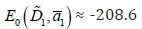meV. It should be emphasized that the criterion (8) of the applicability of the variational method for the calculation of the biexciton binding energy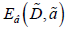is fulfilled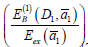≅0.07. At larger distances D between the surfaces ofthe biexciton breaks down into two excitons (consisting of spatially separated electrons and holes), localized over QD surfaces (Figure 1). Thus, a biexciton can be formed in a nano system where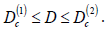Furthermore, a biexciton can exist only at temperatures lower than the critical temperature Tc = 81.6 K. In the aluminum oxide monocrystal with the binding energy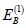is almost 12 times as large.

As follows form the results of the variational calculations, the major contribution to the biexciton binding energy is from the energy of exchange interaction of electrons and holes, which by far surpasses that from their Coulomb interaction (i.e. the ratio ≤ 0.11). Since the calculations of the biexciton ground state binding energy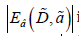in the nano system are variational, the values of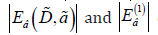can be somewhat underestimated.

#### Biexciton in Quasicrystal

In a NHS (mean radius of QD 1 a = 3.18 nm, and the distance between the surfaces of aluminum oxide QD D1 = 2.93 nm) at temperatures below the critical value Tc = 81.6K (which corresponds to the biexciton binding energy Eâ = 7.03 meV) an “exciton molecule” can be formed (biexciton consisting of spatially separated electrons and holes). Acting as “exciton molecules” in such an NHS are the quantum dots of aluminum oxide, with excitons (consisting of spatially separated electrons and holes) localized over their surfaces. As our variational calculations show, the interaction of the excitons with the surfaces of QD (“intramolecular” interaction) is much stronger than that between quantum dots (“intermolecular” interaction). Due to the translational symmetry of such an NHS of QD, it permits propagation of electronic excitation in the form of biexcitons.

The energy level of the biexciton(7) turns, in this case, into a biexciton energy band with the width(whereis the translational mass of the exciton of spatially separated electron and hole). Quantitative assessment of the width of the biexciton band yields the value of about 3 meV (which corresponds to the temperature of 35 K). In such an NHS the energy band of biexciton states is located below the bandgap of aluminum oxide NHS by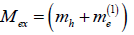is the translational mass of the exciton of spatially separated electron and hole). Quantitative assessment of the width of the biexciton band yields the value of about 3 meV (which corresponds to the temperature of 35 K). In such an NHS the energy band of biexciton states is located below the bandgap of aluminum oxide NHS by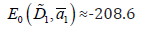The position of this energy band is governed both by the mean radiusand the distance D1 between QD surfaces. Thus, by varying these parameters of the NHS, one can purposefully control the position of the energy band of biexciton states.

At higher temperatures (Т ≥ Tc), a phase transition of the nano system can occur from the biexciton to exciton state. At a constant concentration of excitons (i.e. constant concentration of QD) and temperatures Т lower than Tc, one can expect a new luminescence band shifted from the exciton band by the value of the biexciton binding energy â E . This new band disappears at higher temperatures (Т ≥ Tc). At a constant temperature below critical (Т< Tc), an increase in exciton concentration (i.e. in QD concentration) brings about weakening of the exciton luminescence band and strengthening of the biexciton one.

#### Conclusion

Thus it has been shown that the exciton quasimolecule formation in a NHS made up of aluminum oxide quantum dots (QD) of the mean radiusis of threshold character and can occur in a nano system where the distance D between the surfaces of QD is given by the conditionhave demonstrated that in such a NHS (with the mean radius 1 a = 3.18 nm and the distance between surfaces of aluminum oxide QD D1 = 2.93 nm) at temperatures lower than the critical temperature Tc = 81.6K (which corresponds to the exciton quasimolecule binding energy â E = 7.03 meV) an ,,exciton molecule“ (a biexciton consisting of spatially separated electrons and holes) can be formed. Acting as “exciton molecules” in the NHS are aluminum oxide quantum dots with excitons (consisting of spatially separated electrons and holes) localizing over their surfaces. The interaction of the excitons with the QD surfaces (“intramolecular” interaction) is shown to be much stronger than that between quantum dots (“intermolecular” interaction).

We have also shown that the position of the biexciton band in the NHS depends both on the QD mean radius 1 a and the distance D1 between QD surfaces. By varying these parameters, one can purposefully control the position of the energy band of biexciton states in the NHS. With increase in temperature above the threshold (Т ≥ Tc), a phase transition can occur from the biexciton to exciton state. It has been found that at a constant concentration of excitons (i.e. constant concentration of QD) and temperatures Т below Tc, one can expect a new luminescence band shifted from the exciton band by the value of the biexciton binding energy â E . This new band disappears at higher temperatures (Т ≥ Tc). At a constant temperature below Tc, an increase in exciton concentration (i.e. in QD concentration) brings about weakening of the exciton luminescence band and strengthening of the biexciton one.

Thus, NHS made up of “exciton molecules” are of considerable interest both physically and practically as novel nanomaterials for nano-optoelectronics [34-42].

None.

#### Conflict of Interest

No conflict of interest.

Article Details
Article Details
Citation
Keywords
Scroll to Top